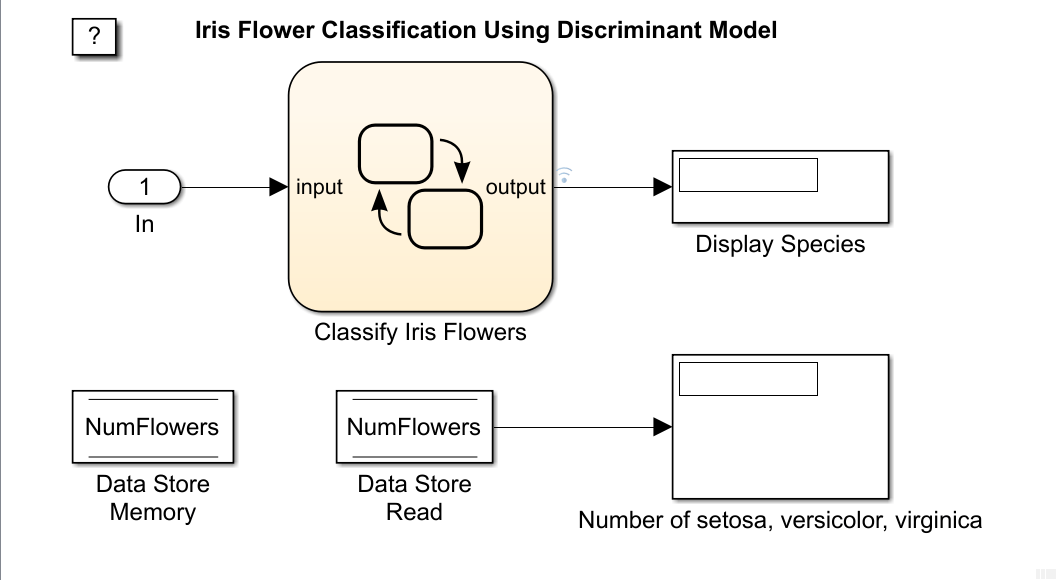# Predict Class Labels Using Stateflow

This example shows how to use a Stateflow® chart for label prediction. The example trains a discriminant analysis model for the Fisher iris data set by using `fitcdiscr`, and defines a function for code generation that loads the trained model and predicts labels for new data. The Stateflow chart in this example accepts streaming data and predicts labels using the function you define.

Fisher's iris data set, which is included in Statistics and Machine Learning Toolbox™, contains species (`species`) and measurements (`meas`) on sepal length, sepal width, petal length, and petal width for 150 iris specimens. The data set contains 50 specimens from each of three species: setosa, versicolor, and virginica.

Load the Fisher iris data set.

```load fisheriris ```

Convert `species` to an index vector where 1, 2, and 3 correspond to setosa, versicolor, and virginica, respectively.

```species = grp2idx(species); ```

Partition the data into a training set and a test set.

```rng('default') % For reproducibility idx1 = randperm(150,75)'; idx2 = setdiff((1:150)',idx1); X = meas(idx1,:); Y = species(idx1,:); trainX = meas(idx2,:); trainY = species(idx2,:); ```

Use `trainX` and `trainY` to train a model, and use `X` and `Y` to test the trained model.

Train a quadratic discriminant analysis model.

```Mdl = fitcdiscr(trainX,trainY,'DiscrimType','quadratic'); ```

`Mdl` is a `ClassificationDiscriminant` model. At the command line, you can use `Mdl` to make predictions for new observations. However, you cannot use `Mdl` as an input argument in a function for code generation. Prepare `Mdl` to be loaded within the function by using `saveLearnerForCoder`.

```saveLearnerForCoder(Mdl,'DiscrIris'); ```

`saveLearnerForCoder` compacts `Mdl` and saves it in the MAT-file `DiscrIris.mat`.

To display the predicted species in the display box of the Stateflow® model, define an enumeration class by using a `classdef` block in the MATLAB file `IrisSpecies.m`.

```classdef IrisSpecies < Simulink.IntEnumType enumeration Setosa(1) Versicolor(2) Virginica(3) end end ```

For details about enumerated data, see Define Enumerated Data Types (Stateflow).

Define a function named `mypredict.m` that predicts the iris species from new measurement data by using the trained model. The function should:

• Include the code generation directive `%#codegen` somewhere in the function.

• Accept iris measurement data. The data must be consistent with X except for the number of rows.

• Load `DiscrIris.mat` using `loadLearnerForCoder`.

• Return predicted iris species.

```function label = mypredict(X) %#codegen %MYPREDICT Predict species of iris flowers using discriminant model % mypredict predicts species of iris flowers using the compact % discriminant model in the file DiscrIris.mat. Rows of X correspond to % observations and columns correspond to predictor variables. label is % the predicted species. mdl = loadLearnerForCoder('DiscrIris'); labelTemp = predict(mdl,X); label = IrisSpecies(labelTemp); end ```

Open the Simulink® model `sf_countflowers.slx`.

```sfName = 'sf_countflowers'; open_system(sfName); ```The figures display the Simulink® model and the flow graph contained in the Stateflow® chart. When the input node detects measurement data, it directs the data into the chart. The chart then predicts a species of iris flower and counts the number of flowers for each species. The chart returns the predicted species to the workspace and displays the species within the model, one at a time. The data store memory block `NumFlowers` stores the number of flowers for each species.

The chart expects to receive input data as a structure array called `fisheririsInput` containing these fields:

• `time` - The points in time at which the observations enter the model. In the example, the duration includes the integers from 0 through 74. The orientation of `time` must correspond to the observations in the predictor data. So, for this example, `time` must be a column vector.

• `signals` - A 1-by-1 structure array describing the input data and containing the fields `values` and `dimensions`. The `values` field is a matrix of predictor data. The `dimensions` field is the number of predictor variables.

Create an appropriate structure array for iris flower measurements.

```fisheririsInput.time = [0:74]'; fisheririsInput.signals.dimensions = 4; fisheririsInput.signals.values = X; ```

You can change the name from fisheririsInput, and then specify the new name in the model. However, Stateflow® expects the structure array to contain the described field names. For more details, see Loading Data Structures to Root-Level Inputs (Simulink).

Simulate the model.

```sim(sfName) ```The figure shows the model after it processes all observations in `fisheririsInput`, one at a time. The predicted species of `X(75,:)` is virginica. The number of setosa, versicolor, and virginica in `X` is 22, 22, and 31, respectively.

The variable `logsout` appears in the workspace. `logsout` is a `SimulinkData.Dataset` object containing the predicted species. Extract the predicted species data from the simulation log.

```labelSF = logsout.getElement(1).Values.Data; ```

Predict species at the command line using `predict`.

```labelCMD = predict(Mdl,X); ```

Compare the predicted species returned by `sf_countflowers` to those returned by calling `predict` at the command line.

```isequal(labelCMD,labelSF) ```
```ans = logical 1 ```

`isequal` returns logical 1 (`true`) if all the inputs are equal. This comparison confirms that `sf_countflowers` returns the expected results.

If you also have a Simulink® Coder™ license, then you can generate C code from `sf_countflowers.slx` in Simulink® or from the command line using `rtwbuild` (Simulink Coder). For more details, see Generate C Code for a Model (Simulink Coder).